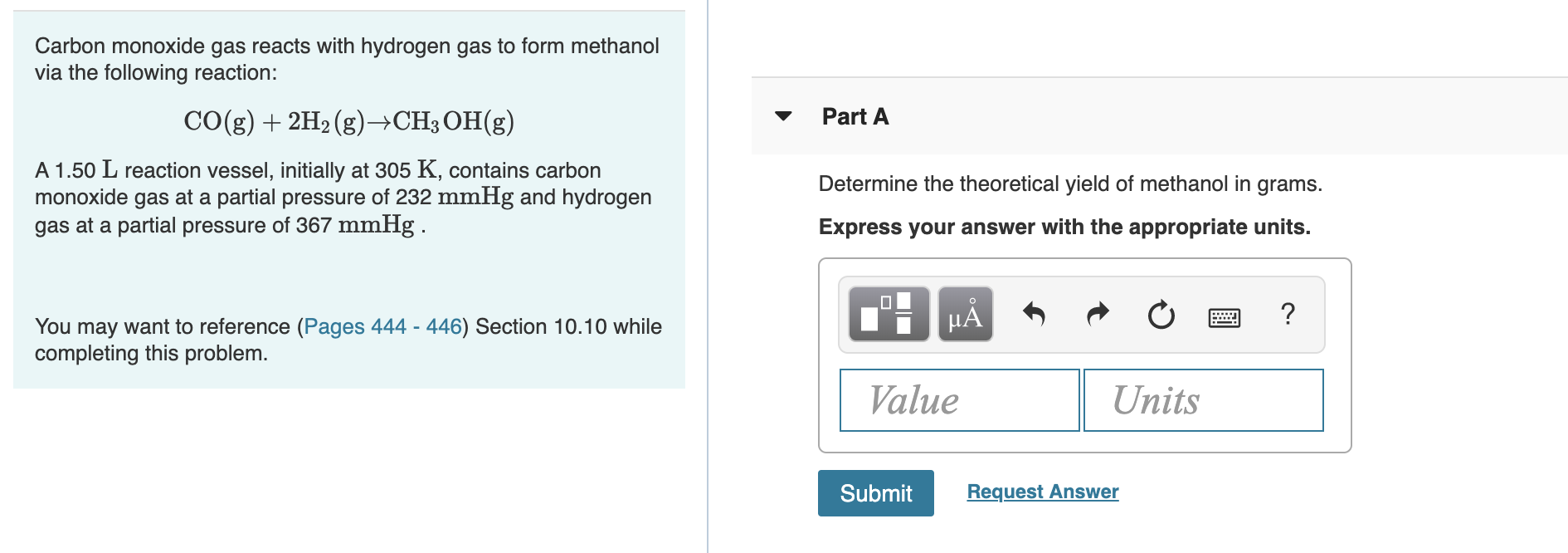# Carbon monoxide gas reacts with hydrogen gas to form methanolvia the following reaction:CO(g)2H2 (g)->CH3 OH(g)Part AA 1.50 L reaction vessel, initially at 305 K, contains carbonmonoxide gas at a partial pressure of 232 mmHg and hydrogengas at a partial pressure of 367 mmHgDetermine the theoretical yield of methanol in grams.Express your answer with the appropriate units.?You may want to reference (Pages 444 - 446) Section 10.10 whilecompleting this problemValueUnitsRequest AnswerSubmit

Question
28 viewshelp_outlineImage TranscriptioncloseCarbon monoxide gas reacts with hydrogen gas to form methanol via the following reaction: CO(g)2H2 (g)->CH3 OH(g) Part A A 1.50 L reaction vessel, initially at 305 K, contains carbon monoxide gas at a partial pressure of 232 mmHg and hydrogen gas at a partial pressure of 367 mmHg Determine the theoretical yield of methanol in grams. Express your answer with the appropriate units. ? You may want to reference (Pages 444 - 446) Section 10.10 while completing this problem Value Units Request Answer Submit fullscreen
check_circle

star
star
star
star
star
1 Rating
Step 1

The number of moles (n1) of CO (g) inside the 1.50 L reaction vessel at 305 K is calculated as shown in equation (1) where R is the gas constant and P1 is the partial pressure of CO. The values for R and P1 are substituted in equation (1). The number of moles (n1) of CO (g) inside the 1.50 L reaction vessel at 305 K is 0.018 mol.

Step 2

The number of moles (n2) of H2 (g) inside the 1.50 L reaction vessel at 305 K is calculated as shown in equation (2) where R is the gas constant and P2 is the partial pressure of H2. The values for R and P1 are substituted in equation (2). The number of moles (n2) of H2 (g) inside the 1.50 L reaction vessel at 305 K is 0.029 mol.

Step 3

According to the given chemical reaction, 0.018 mol of CO (g) require 0.036 mol of H2 (g) thus, H2 (g) is the limiting reagent and the amount of formation of CH3OH depends upon th...

### Want to see the full answer?

See Solution

#### Want to see this answer and more?

Solutions are written by subject experts who are available 24/7. Questions are typically answered within 1 hour.*

See Solution
*Response times may vary by subject and question.
Tagged in

### Gas laws